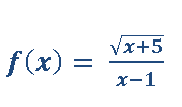Clutch Prep is now a part of Pearson
Ch.1: Pre-Calc (Part 1)WorksheetSee all chapters

# Computing Domain

See all sections
Sections
Representation of a Function
Word Problems as Functions
Types of Functions
Asymptotes
Rational Functions
Computing Domain
Domain & Range

Concept #1: Intro

Concept #2

Practice: Find the domain for each of the following function,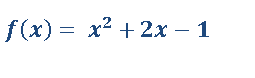Practice: Find the domain for each of the following function,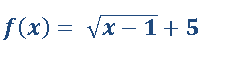Practice: Find the domain for each of the following function,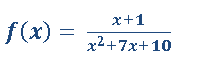Practice: Find the domain for each of the following function,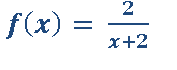Practice: Find the domain for each of the following function,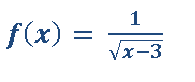Practice: Find the domain for each of the following function,